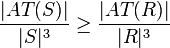# Associating fraction in subring of finite non-associative ring is at least as much as in whole ring

## Statement

Suppose$R$ is a finite Non-associative ring (?) (i.e.,$R$ is a not necessarily associative ring whose underlying set is finite). Suppose$S$ is a subring of$R$. Then, the associating fraction of$S$ is at least as much as that of$R$.

In symbols, if$AT(R) := \{ (x,y,z) \in R^3 \mid (x * y) * z = x * (y * z) \}$ and$AT(S) = S^3 \cap CP(R)$, then:$\frac{|AT(S)|}{|S|^3} \ge \frac{|AT(R)|}{|R|^3}$

In fact, the result also holds if$S$ is simply an additive subgroup of$R$ and not a subring.

## Proof

The proof follows from facts (1) and (2).